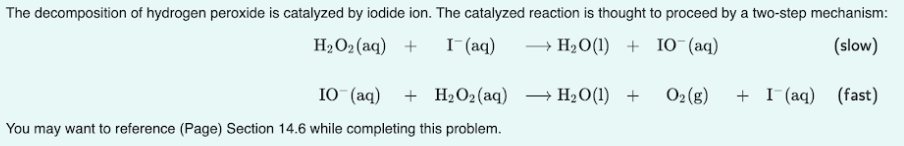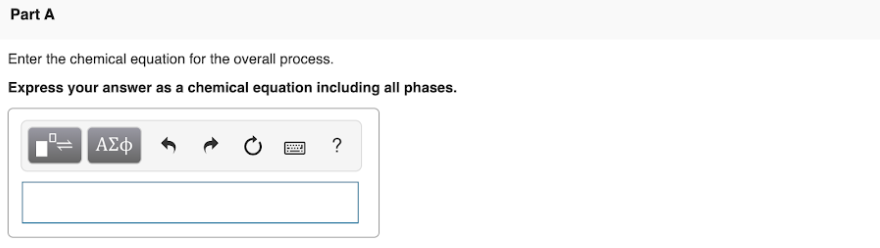# The decomposition of hydrogen peroxide is catalyzed by iodide ion. The catalyzed reaction is thought to proceed by a two-step mechanism: H2O2(aq) + I^-(aq) → H2O(l) + IO^-(aq) (slow) IO^-(aq) + H2O2(aq) → H2O(l) + O2(g) + I^-(aq) (fast) You may want to reference (page) Section 14.6 while completing this problem. Enter the chemical equation for the overall process. Express your answer as a chemical equation including all phases.